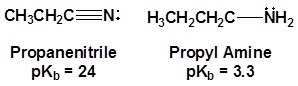# Nitrile Properties

$$\newcommand{\vecs}{\overset { \rightharpoonup} {\mathbf{#1}} }$$ $$\newcommand{\vecd}{\overset{-\!-\!\rightharpoonup}{\vphantom{a}\smash {#1}}}$$$$\newcommand{\id}{\mathrm{id}}$$ $$\newcommand{\Span}{\mathrm{span}}$$ $$\newcommand{\kernel}{\mathrm{null}\,}$$ $$\newcommand{\range}{\mathrm{range}\,}$$ $$\newcommand{\RealPart}{\mathrm{Re}}$$ $$\newcommand{\ImaginaryPart}{\mathrm{Im}}$$ $$\newcommand{\Argument}{\mathrm{Arg}}$$ $$\newcommand{\norm}{\| #1 \|}$$ $$\newcommand{\inner}{\langle #1, #2 \rangle}$$ $$\newcommand{\Span}{\mathrm{span}}$$ $$\newcommand{\id}{\mathrm{id}}$$ $$\newcommand{\Span}{\mathrm{span}}$$ $$\newcommand{\kernel}{\mathrm{null}\,}$$ $$\newcommand{\range}{\mathrm{range}\,}$$ $$\newcommand{\RealPart}{\mathrm{Re}}$$ $$\newcommand{\ImaginaryPart}{\mathrm{Im}}$$ $$\newcommand{\Argument}{\mathrm{Arg}}$$ $$\newcommand{\norm}{\| #1 \|}$$ $$\newcommand{\inner}{\langle #1, #2 \rangle}$$ $$\newcommand{\Span}{\mathrm{span}}$$$$\newcommand{\AA}{\unicode[.8,0]{x212B}}$$

The electronic structure of nitriles is very similar to that of an alkyne with the main difference being the presence of a set of lone pair electrons on the nitrogen. Both the carbon and the nitrogen are sp hydridized which leaves them both with two p orbitals which overlap to form the two $$\pi$$ bond in the triple bond. The R-C-N bond angle in and nitrile is 180° which give a nitrile functional group a linear shape.The lone pair electrons on the nitrogen are contained in a sp hybrid orbital which makes them much less basic and an amine. The 50% character of an sp hybrid orbital close to the nucleus and therefore less basic compared to other nitrogen containing compounds such as amines.The presence of an electronegative nitrogen causes nitriles to be very polar molecules. Consequently, nitriles tend to have higher boiling points than molecules with a similar size.## Contributors

Nitrile Properties is shared under a CC BY-NC-SA 4.0 license and was authored, remixed, and/or curated by LibreTexts.